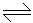# Chemical Engineering - Chemical Reaction Engineering

### Exercise :: Chemical Reaction Engineering - Section 9

46.

Fluidised bed reactor is characterised by

 A. uniformity of temperature. B. comparatively smaller equipment. C. very small pressure drop. D. absence of continuous catalyst regeneration facility.

Explanation:

No answer description available for this question. Let us discuss.

47.

In which of the following gaseous phase reversible reactions, the product yield can not be increased by the application of high pressure ?

 A. N2 + O22NO B. PCl3 + Cl2PCl5 C. N + 3H22NH3 D. 2SO2 + O22SO3

Explanation:

No answer description available for this question. Let us discuss.

48.

Space time equals the mean residence time

 A. when the density of the reaction mixture is constant. B. for large diameter tubular reactor. C. for narrow diameter tubular reactor. D. for CSTR.

Explanation:

No answer description available for this question. Let us discuss.

49.

A liquid decomposes by irreversible first order kinetics and the half life period of this reaction is 8 minutes. The time required for 75% conversion of the liquid will be __________ minutes.

 A. 4 B. 8 C. 12 D. 16

Explanation:

No answer description available for this question. Let us discuss.

50.

Rate constant 'k' and absolute temperature 'T' are related by collision theory (for bimolecular) as

 A. k ∝ T1.5 B. k ∝ exp(-E/RT) C. k ∝ T D. k ∝ T